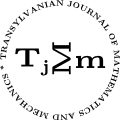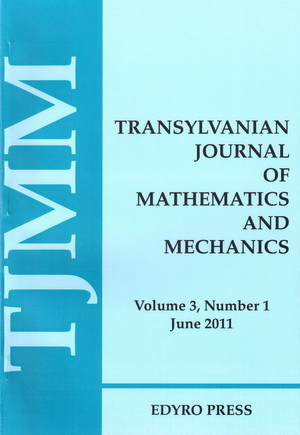ISSN: 2067-239X
ISSN(on-line): 2067-239X

Indexed in:
Mathematical Reviews
Zentralblatt MATH
EBSCO

Front cover# Volume 7 (2015), Number 2

## On Subclass of Harmonic Univalent Functions Deﬁned by Generalized Salagean Operator and Ruscheweyh Operator

### Author(s): MOHAMMAD AL-KASEASBEH and MASLINA DARUS

Abstract: A subclass of complex-valued harmonic univalent function deﬁned by generalized Salagean operator and Ruscheweyh operator is introduced. Coefficient bounds, distortion theorem, and other properties of this class are obtained.

## A Mathematical Model to Extend the Theory of Relativity when the Velocities of the Mass are Larger than the Velocity of the Light c and its Possible Application in Cosmology

### Author(s): MALVINA BAICA and MIRCEA CARDU

Abstract: In this paper we analyze the subject in the title accepting that the Mathematical model from the Theory of Relativity (RT) corresponding to the velocity $v is also valid to the case $v>c$. In the same time we apply the method to solve the equations of type ${y}^{2}±\phi =0$ in their simplest form ${y}^{2}±1=0$ developed in  and . Here we assign the “dual” solutions for $v and $v>c$ respectively and the “bipolar” solutions for the two forms of the Mass in the Universe called Matter (MA) and Antimatter (AM), respectively.

## Global Existence of Solution for Reaction Diﬀusion Systems with a Full Matrix

### Author(s): K. BOUKERRIOUA

Abstract: The aim of this paper is to study the global existence in time of solutions for some class of reaction-diffusion systems with full matrix.Our techniques are based on invariant regions and Lyapunov functional methods.Our goal is to show, under suitable assumptions, that the proposed model have a global solution for a large class of the functions $f$ and $g$.

## An Integral Equation from Physics - A Synthesis Survey - Part II

### Author(s): MARIA DOBRIȚOIU

Abstract: This part of the synthesis survey on the study of the integral equation from physics:

 $x\left(t\right)={\int }_{a}^{b}K\left(t,s,x\left(s\right),x\left(a\right),x\left(b\right)\right)ds+f\left(t\right),\phantom{\rule{1em}{0ex}}t\in \left[a,b\right].$
contains the results concerning the continuous data dependence and the differentiability of its solution. This part ends with some examples.

## Approximate Solution of a Nonlinear Fractional Ordinary Differential Equation by DGJ Method

### Author(s): YIYING FENG and YUE HU

Abstract: Applying the new iterative method (DGJM), which have been used to handle the nonlinear models, we investigate the approximate analytical solutions for a nonlinear fractional ordinary differential equation (FODE), where the fractional derivatives are considered in Caputo sense. On the process of dealing with nonlinear terms, we particularly employ Taylor series expansion to obtain the analytical solutions.

## The generalized $\left(s,t\right)$-Fibonacci and Fibonacci matrix sequences

### Author(s): AHMET İPEK, KAMIL ARI and RAMAZAN TÜRKMEN

Abstract: In this paper, we study the generalizations of the $\left(s,t\right)$-Fibonacci and Lucas sequences and the $\left(s,t\right)$-Fibonacci and Lucas matrix sequences. We present relationship between the $\left(s,t\right)$-Fibonacci matrix and generalized Fibonacci matrix sequences. Binet’s formula for the generalized $\left(s,t\right)$-Fibonacci matrix sequence is derived. We establish several identites for the generalized $\left(s,t\right)$-Fibonacci and Fibonacci matrix sequence. We give some partial sum formulas for the generalized $\left(s,t\right)$-Fibonacci and Fibonacci matrix sequence. Also, we ﬁnd out relationship between the $\left(s,t\right)$-Fibonacci matrix sequence and the famous Bernoulli numbers.